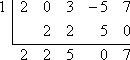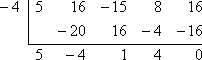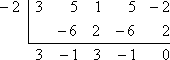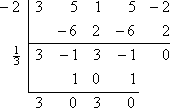Search

The Factor Theorem

The Factor Theorem is a result of the Remainder Theorem, and is based on the same reasoning. If you haven't read the lesson on the Remainder Theorem, review that topic first, and then return here.As the Remainder Theorem points out, if you divide a polynomial p(x) by a factor x  a of that polynomial, then you will get a zero remainder. Let's look again at that Division Algorithm expression of the polynomial:

Advertisement

p(x) = (x  a)q(x) + r(x)

If x  a is indeed a factor of p(x), then the remainder after division by x  a will be zero. That is:

p(x) = (x  a)q(x)

In terms of the Remainder Theorem, this means that, if x  a is a factor of p(x), then the remainder, when we do synthetic division by
x = a, will be zero.

The point of the Factor Theorem is the reverse of the Remainder Theorem: If you synthetic-divide a polynomial by x = a and get a zero remainder, then, not only is x = a a zero of the polynomial (courtesy of the Remainder Theorem), but x  a is also a factor of the polynomial (courtesy of the Factor Theorem).

Just as with the Remainder Theorem, the point here is not to do the long division of a given polynomial by a given factor. This Theorem isn't repeating what you already know, but is instead trying to make your life simpler. When faced with a Factor Theorem exercise, you will apply synthetic division and then check for a zero remainder.

• Use the Factor Theorem to determine whether x  1 is a factor of

f (x) = 2x4 + 3x2  5x + 7.

For x  1 to be a factor of  f (x) = 2x4 + 3x2  5x + 7, the Factor Theorem says that x = 1 must be a zero of  f (x). To test whether x  1 is a factor, I will first set x  1 equal to zero and solve to find the proposed zero, x = 1. Then I will use synthetic division to divide f (x) by x = 1. Since there is no cubed term, I will be careful to remember to insert a "0" into the first line of the synthetic division to represent the omitted power of x in 2x4 + 3x2  5x + 7:Since the remainder is not zero, then the Factor Theorem says that:

x  1 is not a factor of f (x).

• Using the Factor Theorem, verify that x + 4 is a factor of

f (x) = 5x4 + 16x3  15x2 + 8x + 16.

If x + 4 is a factor, then (setting this factor equal to zero and solving) x = 4 is a root. To do the required verification, I need to check that, when I use synthetic division on  f (x), with x = 4, I get a zero remainder:The remainder is zero, so the Factor Theorem says that:

x + 4 is a factor of 5x4 + 16x3  15x2 + 8x + 16.

In practice, the Factor Theorem is used when factoring polynomials "completely". Rather than trying various factors by using long division, you will use synthetic division and the Factor Theorem. Any time you divide by a number (being a potential root of the polynomial) and get a zero remainder in the synthetic division, this means that the number is indeed a root, and thus "x minus the number" is a factor. Then you will continue the division with the resulting smaller polynomial, continuing until you arrive at a linear factor (so you've found all the factors) or a quadratic (to which you can apply the Quadratic Formula).

• Using the fact that 2 and 1/3 are zeroes of  f (x) = 3x4 + 5x3 + x2 + 5x  2, factor the polynomial completely.   Copyright © Elizabeth Stapel 2002-2011 All Rights Reserved

If x = 2 is a zero, then x + 2 = 0, so x + 2 is a factor. Similarly, if x = 1/3 is a zero, then x  1/3 = 0, so x  1/3 is a factor. By giving me two of the zeroes, they have also given me two factors: x + 2 and x  1/3.

Since I started with a fourth-degree polynomial, then I'll be left with a quadratic once I divide out these two given factors. I can solve that quadratic by using the Quadratic Formula or some other method.

The Factor Theorem says that I don't have to do the long division with the known factors of x + 2 and x  1/3. Instead, I can use synthetic division with the associated zeroes 2 and 1/3. Here is what I get when I do the first division with x = 2:The remainder is zero, which is expected because they'd told me at the start that 2 was a known zero of the polynomial. Rather than starting over again with the original polynomial, I'll now work on the remaining polynomial factor of 3x3  x2 + 3x  1 (from the bottom line of the synthetic division). I will divide this by the other given zero, x = 1/3:This leaves me with the quadratic 3x2 + 3, which I can solve:

3x2 + 3 = 0
3(x2 + 1) = 0
x2 + 1 = 0
x2 = 1
x = ± i

If the zeroes are x = i and x = i, then the factors are x  (i) and x  (i), or x + i and x  i. I need to remember that I divided off a "3" when I solved the quadratic; it is still part of the polynomial, and needs to be included as a factor. Then the fully-factored form is:

3x4 + 5x3 + x2 + 5x  2 = 3(x + 2)(x  1/3)(x + i)(x  i)

Top  |  Return to Index

 Cite this article as: Stapel, Elizabeth. "The Factor Theorem." Purplemath. Available from     https://www.purplemath.com/modules/factrthm.htm. Accessed [Date] [Month] 2016

Study Skills Survey

Tutoring from Purplemath
Find a local math tutor

 Copyright © 2021  Elizabeth Stapel   |   About   |   Terms of Use   |   Linking   |   Site Licensing Contact Us